# AP Board 7th Class Maths Solutions Chapter 2 Fractions, Decimals and Rational Numbers Review Exercise

SCERT AP 7th Class Maths Solutions Pdf Chapter 2 Fractions, Decimals and Rational Numbers Review Exercise Questions and Answers.

## AP State Syllabus 7th Class Maths Solutions 2nd Lesson Fractions, Decimals and Rational Numbers Review Exercise

Question 1.
Write the following fractions in ascending order.
(i) $$\frac{3}{2}, \frac{5}{2}, \frac{1}{2}, \frac{17}{2}, \frac{9}{2}$$
Given $$\frac{3}{2}, \frac{5}{2}, \frac{1}{2}, \frac{17}{2}, \frac{9}{2}$$
Given fractions are like fractions.

So, we should arrange the numerators in ascending order.
$$\frac{1}{2}<\frac{3}{2}<\frac{5}{2}<\frac{9}{2}<\frac{17}{2}$$

Ascending order: $$\frac{1}{2}<\frac{3}{2}<\frac{5}{2}<\frac{9}{2}<\frac{17}{2}$$

(ii) $$\frac{6}{5}, \frac{11}{10}, \frac{19}{5}, \frac{7}{10}, \frac{5}{10}$$
Given $$\frac{6}{5}, \frac{11}{10}, \frac{19}{5}, \frac{7}{10}, \frac{5}{10}$$
Given fractions are not like fractions. So, first we have to convert them into like fractions.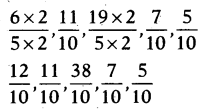Now arrange them in ascending order according to their numerators.
$$\frac{5}{10}<\frac{7}{10}<\frac{11}{10}<\frac{12}{10}<\frac{38}{10}$$
Ascending order: $$\frac{5}{10}<\frac{7}{10}<\frac{11}{10}<\frac{12}{10}<\frac{38}{10}$$

(iii) $$\frac{8}{3}, \frac{7}{6}, 3 \frac{1}{4}, \frac{5}{3}, \frac{11}{4}$$
Given $$\frac{8}{3}, \frac{7}{6}, 3 \frac{1}{4}, \frac{5}{3}, \frac{11}{4}$$
$$\frac{8}{3}, \frac{7}{6}, \frac{13}{4}, \frac{5}{3}, \frac{11}{4}$$
We have to find the LCM of denominators to convert them into equal fraction.

LCM of numerators is 12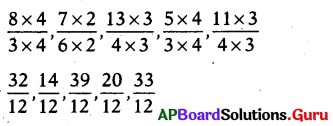Now arrange the like fractions in ascending order according to their numerators.
$$\frac{14}{12}<\frac{20}{12}<\frac{32}{12}<\frac{33}{12}<\frac{39}{12}$$
Ascending order :
$$\frac{14}{12}<\frac{20}{12}<\frac{32}{12}<\frac{33}{12}<\frac{39}{12}$$Question 2.
Calculate the following.
(i) $$\frac{3}{5}+\frac{7}{4}$$
Given $$\frac{3}{5}+\frac{7}{4}=\frac{12+35}{20}=\frac{47}{20}$$

(ii) $$\frac{5}{6}+\frac{7}{12}$$
Given $$\frac{5}{6}+\frac{7}{12}$$
= $$\frac{5 \times 2}{6 \times 2}+\frac{7}{12}$$
= $$\frac{10}{12}+\frac{7}{12}$$
= $$\frac{10+7}{12}=\frac{17}{12}=1 \frac{5}{12}$$

(iii) 1$$\frac{7}{8}-\frac{1}{5}$$
Given 1$$\frac{7}{8}-\frac{1}{5}$$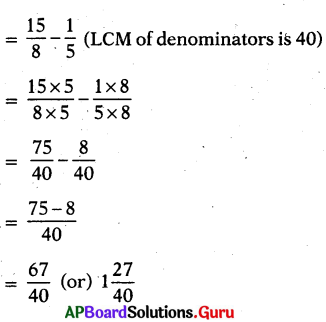(iv) 4$$\frac{1}{2}$$ + 3$$\frac{1}{3}$$
Given 4$$\frac{1}{2}$$ + 3$$\frac{1}{3}$$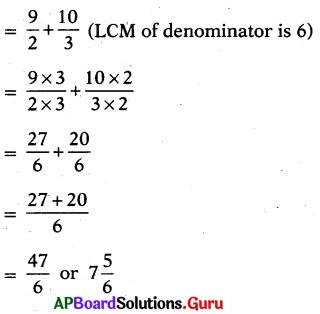Question 3.
Simplify the following.
(i) $$\frac{1}{4}$$ of 3
Given $$\frac{1}{4}$$ of 3
= $$\frac{1}{4} \times \frac{3}{1}=\frac{3}{4}$$

(ii) $$\frac{5}{8}$$ of $$\frac{2}{3}$$
Given $$\frac{5}{8}$$ of $$\frac{2}{3}$$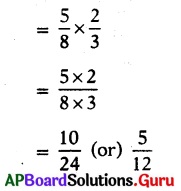(iii) $$\frac{15}{4} \times 2 \frac{1}{7}$$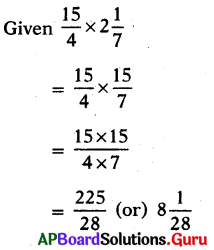(iv) 3$$\frac{1}{3}$$ × 2$$\frac{2}{5}$$
Given 3$$\frac{1}{3}$$ × 2$$\frac{2}{5}$$
= $$\frac{10}{3} \times \frac{12}{5}$$
= $$\frac{120}{15}=\frac{8}{1}$$ = 8Question 4.
Calculate the following.
(i) $$\frac{3}{4}$$ ÷ 3
Given $$\frac{3}{4}$$ ÷ 3
= $$\frac{3}{4}$$ ÷ $$\frac{3}{1}$$

We know reciprocial of $$\frac{3}{1}$$ is $$\frac{1}{3}$$
= $$\frac{3}{4} \times \frac{1}{3}$$
= $$\frac{3}{12}$$ (or) $$\frac{1}{4}$$

(ii) 8 ÷ 2$$\frac{1}{7}$$
Given 8 ÷ 2$$\frac{1}{7}$$
= 8 ÷ 2$$\frac{15}{7}$$

We know reciprocial of $$\frac{15}{7}$$ is $$\frac{7}{15}$$
= 8 × $$\frac{7}{15}$$
= $$\frac{8 \times 7}{15}$$
= $$\frac{56}{15}$$ (OR) 3$$\frac{11}{15}$$

(iii) $$\frac{12}{7} \div \frac{2}{7}$$
Given $$\frac{12}{7} \div \frac{2}{7}$$
We know reciprocial of $$\frac{12}{7} \div \frac{2}{7}$$
= $$\frac{12}{7} \times \frac{7}{2}$$
= $$\frac{12 \times 7}{7 \times 2}=\frac{84}{14}=\frac{6}{1}$$ = 6
(iv) 5$$\frac{1}{2}$$ ÷ 2$$\frac{9}{11}$$
Given 5$$\frac{1}{2}$$ ÷ 2$$\frac{9}{11}$$
= $$\frac{11}{2} \div \frac{31}{11}$$
We know reciprocial of $$\frac{31}{11}$$ is $$\frac{11}{31}$$
= $$\frac{11}{2} \times \frac{11}{31}$$
= $$\frac{121}{62}$$ (OR) 1$$\frac{59}{62}$$